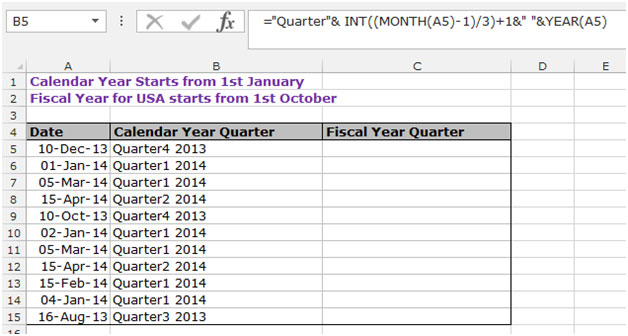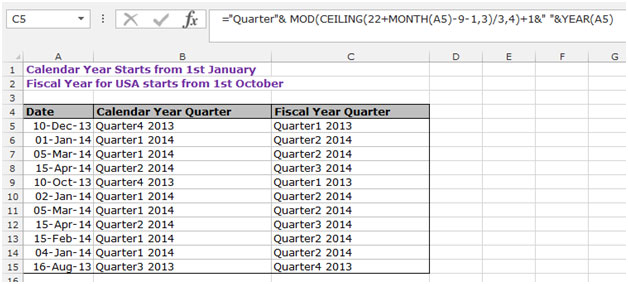How to Calculate the Quarter in Microsoft Excel

In this article we will learn how to calculate the quarter number for Fiscal Year and Calendar Year  in  Microsoft Excel.

To calculate quarter in Excel you can use the formula of “INT”, “MONTH”, “MOD” and “CEILING” function.

INT function is used to return the whole number without decimals. This is better than changing the number of decimal places displayed, which would risk some numbers being rounded up and thus giving an incorrect result.

MOD function is used to returns the remainder after a number is divided by a divisor.

MONTH function is used to return the month in numbers.

CEILING function is used to roundup the number to the nearest multiple of a specified value.

Let’s take an example and understand how to calculate the quarter number.

We have a table of dates, in which we want to return the quarter according to the calendar year and fiscal year.

To calculate excel date quarter for a calendar year:-

• Select the cell B5 and write the formula to calculate the quarter number
• =”Quarter”& INT ((MONTH (A5)-1)/3) +1&” “&YEAR (A5) and press Enter on the keyboard.
• This function will return the Quarter no. according to the calendar which is Quarter 4 2013.
• To return the quarter no. for the rest of the cells, copy the same formula by pressing the key “CTRL+C” and paste it in the range B6:B15 by pressing the key “CTRL+V” on your keyboard.To calculate the quarter number for a fiscal year ending in September:

• Select the cell C5 and write the formula to calculate the quarter number.
• =”Quarter”&MOD(CEILING(22+MONTH(A5)-9-1,3)/3,4)+1&” “&YEAR(A5) and press Enter on the keyboard.
• This function will return the Quarter no. according to the fiscal calendar which is Quarter 1 2013.
• To return the quarter no. for the rest of the cells, copy the same formula by pressing the key “CTRL+C” and paste it in the range C6:C15 by pressing the key “CTRL+V” on your keyboard.This function is useful when you have to do certain analysis based on quarters and compare the data of one quarter with another.

Also, you can choose whether you need the quarter as per the financial year or as per the fiscal year and use the appropriate formula to give you the right quarter numbers for your data.We would love to hear from you, do let us know how we can improve, complement or innovate our work and make it better for you. Write us at info@exceltip.com

## Users are saying about us...

1.I’m sure the tip shown works but I have used a cheap & dirty vlookup on a table that I create. The table has 2 columns, column 1 has numbers 1-12 which represent months, column 2 reads: 1 1 1 2 2 2 3 3 3 4 4 4 I just vlookup the month in whatever source date I’m using to the month in my lookup table and return the column 2 value. Works for me !! J.

•for Quarter Finding, if C2 is my date:

1. =(TRUNC((MONTH(C2)-1)/3))+1
2. =(INT((MONTH(C2)-1)/3))+1
3. =INT(CEILING(MONTH(C2),3)/3)
4. =INT((CEILING(MONTH(C2),3)+1)/3)
5. =LOOKUP(MONTH(C2),{1,4,7,10},{1,2,3,4})
6. =ROUNDUP(MONTH(J2)/3,0)
7. =HLOOKUP(MONTH(J2),{1,2,3,4,5,6,7,8,9,10,11,12;1,1,1,2,2,2,3,3,3,4,4,4},2)
8. =CHOOSE(MONTH(J2),1,1,1,2,2,2,3,3,3,4,4,4)

2.“I also use lookup function, but I do not create extra table with month and quarter numbers. I write it directly into formula, for example:
=HLOOKUP(A1,{1,2,3,4,5,6,7,8,9,10,11,12;1,1,1,2,2,2,3,3,3,4,4,4},2)
where A1 is month number
J.”

3.Simple Solution is to use the lookup function:
for example if you want the quarter for 28/02/2014 in MS Excel simply use the function below:
=”Q”&LOOKUP(MONTH(“28/2/2014″),{1,4,7,10},{1,2,3,4})

4.Hello Maitrey,
My company beguin the fiscal year on 01-11-2014
how can i foun the quarter for any date.

Thnaks

Bernardo

5.Hi Bernardo,

=”Quarter”& MOD(CEILING(22+MONTH(A5)-10-1,3)/3,4)+1&” “&YEAR(A5)

Thanks
Nisha

6.=INT(([@Month]-1)/3)+1
=ROUNDUP([@Month]/3,0)
=CHOOSE([@Month],1,1,1,2,2,2,3,3,3,4,4,4) <- Nice for fiscal quarters

7.for Quarter Finding, if C2 is my date:

1. =(TRUNC((MONTH(C2)-1)/3))+1
2. =(INT((MONTH(C2)-1)/3))+1
3. =INT(CEILING(MONTH(C2),3)/3)
4. =INT((CEILING(MONTH(C2),3)+1)/3)
5. =LOOKUP(MONTH(C2),{1,4,7,10},{1,2,3,4})
6. =ROUNDUP(MONTH(C2)/3,0)
7. =HLOOKUP(MONTH(C2),{1,2,3,4,5,6,7,8,9,10,11,12;1,1,1,2,2,2,3,3,3,4,4,4},2)
8. =CHOOSE(MONTH(C2),1,1,1,2,2,2,3,3,3,4,4,4)

8.Except the fiscal year is incorrect …. October to December 2014 would be Q1 2015, not 2014. The USA FY 2016 is from Oct 2015 – Sep 2016.

#### Categories

Terms and Conditions of use

The applications/code on this site are distributed as is and without warranties or liability. In no event shall the owner of the copyrights, or the authors of the applications/code be liable for any loss of profit, any problems or any damage resulting from the use or evaluation of the applications/code.